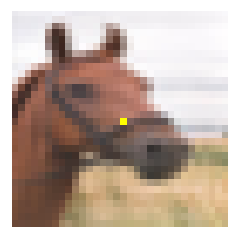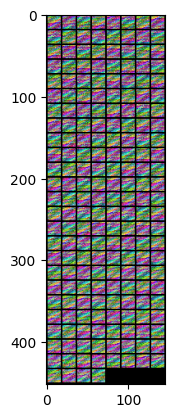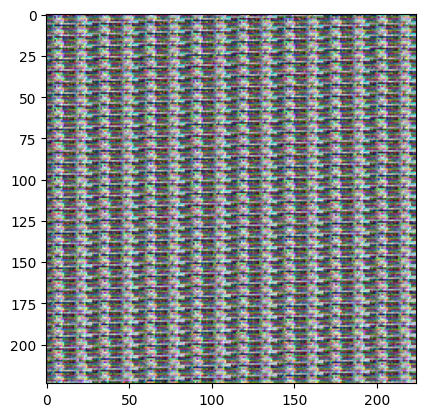# How do I shade a patch

I am dividing an image into patches with the function below:

``````def extract_image_patches(x, kernel, stride=16, dilation=1):
b, c, h, w = x.shape
h2 = math.ceil(h / stride)
w2 = math.ceil(w / stride)
pad_row = (h2 - 1) * stride + (kernel - 1) * dilation + 1 - h
pad_col = (w2 - 1) * stride + (kernel - 1) * dilation + 1 - w

# Extract patches
patches = x.unfold(2, kernel, stride).unfold(3, kernel, stride)
patches = patches.permute(0, 4, 5, 1, 2, 3).contiguous()

return patches.view(b, -1, patches.shape[-2], patches.shape[-1])
``````

For a `224 x 224` image, the above function returns a tensor of shape `(1, 768, 14, 14)`. `768 = (3 x 16 x 16)` for one patch and `(14, 14)` is the height and width. Meaning there are `14` rows and columns of these patches.

Is there a way to change the pixel values of one patch with another function? Below is what I’ve tried so far

``````def shade_patch(patch_list, image):
batch = len(patch_list)
patches = extract_image_patches(image.unsqueeze(0), 16)
pa = patches.repeat(batch, 1, 1, 1)

count = 0

for x in patch_list:
my_patches = np.split(x, len(x)/5

for patch in my_patches:
x_pos, y_pos, r, g, b = patch
pa[count, 0, x_pos, y_pos] = (r / 255.0 - 0.4914) / 0.2023
pa[count, 1, x_pos, y_pos] = (g / 255.0 - 0.4822) / 0.1994
pa[count, 2, x_pos, y_pos] = (b / 255.0 - 0.4465) / 0.2010
count += 1

return pa
``````

Where `patch_list` is a numpy array of 5 elements `([x, y, r, g, b])`. `x` and `y` are the positions of the patch to shade. The positions would range from `0` to `13` in my case since I have `14 x 14` patches. `(r, g, b)` would be the color channels

So if I expect the patch at cordinate `(3, 5)` to have been shaded with `(0, 121, 256)` color combination if I do the following:

``````x = np.array([3, 5, 0, 121, 256])
``````

Your general explanation sounds alright and I’m unsure where you are stuck?
Do you have trouble indexing the patches or do you see an erro running your code?

1 LikeHere is an example of what I want. One pixel has been shaded here. They did this by specifying the x,y cordinate and the colour channels to change to ([x, y, r, g, b]).

In my case, I want to do the same for a patch (16 x16) where I can just specify the patch location (x, y) and replace their color channels (r,g,b) with what I want.

I am not getting any error with the code above. I get a tensor of size [1, 768, 14, 14] which makes sense. However, I tired visualizing if specified patch has been shaded. To do so, I reshaped to (196, 3, 16, 16) but got the following outputYour reshape method is most likely wrong as the output looks interleaved (i.e. the actual indexing might work, but you can’t verify it currently).
Try to reshape the output of your `unfold` method to its original form first without any image manipulations and make sure you are getting the same tensor back.

You are very right sir. Below is the output of the extract_image_patches() function above where the image is being unfolded. This is before any manipulation. It’s probably worth mentioning that I visualized the first one with pytorch’s make_grid functionMaybe this post is helpful, which shows a code snippet how to reshape the unfolded patches back to their original.

Many thanks sir. I’ll check it out

Thank you sir

The post here Fold patches of images back to single image - #3 by agadetsky works for me